Q 1. Find the current in R2 of the given circuit, using the superposition theorem.(A) 16.7 mA
(B) 33.3 mA
(C) 50 mA
(D) 16.6 mA

Q 2. A 120 Ω load is connected across an ideal voltage source with VS = 12 V. The voltage across the load is

(A) 0 V
(B) 12 V
(C) 120 V
(D) Cannot be determined

Q 3. Find the Thevenin equivalent (VTH and RTH) between terminals A and B of the circuit given.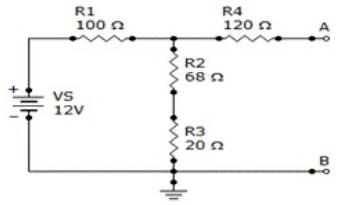(A) 562 mV, 167 Ω
(B) 5.62 V, 167 Ω
(C) 5.62 V, 188 Ω
(D) 562 mV, 188 Ω

Q 4. You cannot convert a voltage source to an equivalent current source, or vice versa.

(A) True
(B) False

Q 5. A certain current source has the values IS = 4 µA and RS = 1.2 MΩ. The values for an equivalent voltage source are

(A) 4.8 μV, 1.2 MΩ
(B) 1 V, 1.2 MΩ
(C) 4.8 V, 4.8 MΩ
(D) 4.8 V, 1.2 MΩ

Q 6. Some circuits require more than one voltage or current source.

(A) True
(B) False

Q 7. Find the Norton circuit, that is, IIN and RN, for the circuit given below.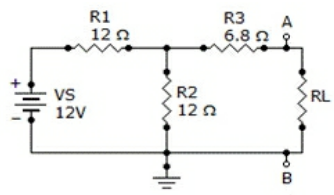(A) 478 mA, 12.8 Ω
(B) 750 mA, 12.8 Ω
(C) 478 mA, 6.8 Ω
(D) 750 mA, 6.8 Ω

Q 8. An ideal current source has zero internal resistance.

(A) True
(B) False

Q 9. Conversions between delta-type and wye-type circuit arrangements are useful in certain specialized applications.

(A) True
(B) False

Q 10. A 2 Ω RL is connected across a voltage source, VS, of 110 V. The source’s internal resistance is 24 Ω. What is the output voltage across the load?

(A) 8.5 V
(B) 85 V
(C) 0 V
(D) 110 V

Q 11. Referring to circuit given, if R1 is changed to a 68 Ω resistor, what will be the current through it?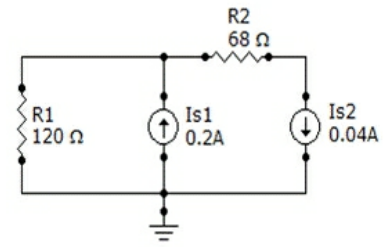(A) 0.16 A
(B) 0.24 A
(C) 0.2 A
(D) 0.04 A

Q 12. Norton’s equivalent current (IN) is an open-circuit current between two points in a circuit.

(A) True
(B) False

Q 13. A 12 mA current source has an internal resistance, RS, of 1.2 kΩ. The equivalent voltage source is

(A) 144 V
(B) 14.4 V
(C) 7.2 V
(D) 72 mV

Q 14. Determine IN for the circuit consisting of VS, R1, R2, and R3 shown in the given circuit.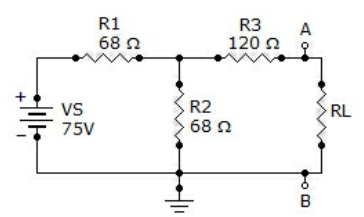(A) 676 mA
(B) 245 mA
(C) 431 mA
(D) 75 mA

Q 15. The Thevenin equivalent voltage (VTH) is the short-circuit voltage between two terminals in a circuit.

(A) True
(B) False

Q 16. In a two-source circuit, one source acting alone produces 12 mA through a given branch. The other source acting alone produces 10 mA in the opposite direction through the same branch. The actual current through the branch is

(A) 22 mA
(B) 12 mA
(C) 10 mA
(D) 2 mA

Q 17. A practical voltage source has a nonzero internal resistance.

(A) True
(B) False

Q 18. Referring to the given circuit, the voltage and current for the load resistor, RL, is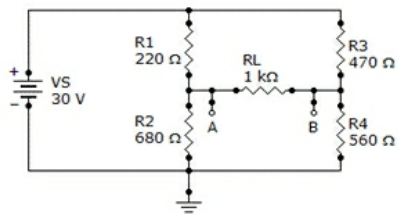(A) 450 mV, 4.5 mA
(B) 4.50 V, 45 mA
(C) 4.50 V, 4.5 mA
(D) 450 mV, 45 mA

Q 19. An ideal voltage source has zero internal resistance.

(A) True
(B) False

Q 20. A 470 Ω RL is connected across a voltage source, VS, of 120 V. The source’s internal resistance, RS, is 12 Ω. What is the output voltage across the load?

(A) 120 V
(B) 0 V
(C) 117 V
(D) 12 V

Q 21. Referring to the given circuit, determine VTH and RTH if a 68 Ω resistor is connected in parallel across R2 and R3.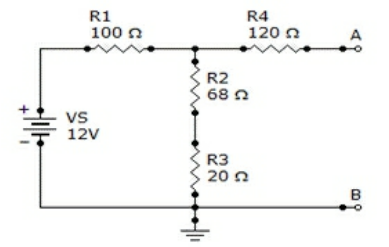(A) 3.3 V, 148 Ω
(B) 330 mV, 148 Ω
(C) 3.3 V, 68 Ω
(D) 330 mV, 68 Ω

Q 22. A certain voltage source has the values VS = 30 V and RS = 6 Ω. The values for an equivalent current source are

(A) 5 A, 6 Ω
(B) 30 A, 6 Ω
(C) 5 A, 30 Ω
(D) 30 A, 5 Ω

Q 23. Transistors act basically as voltage sources.

(A) True
(B) False

Q 24. A 12 V source has an internal resistance of 90 Ω. If a load resistance of 20 Ω is connected to the voltage source, the load power, PL, is

(A) 2.38 mW
(B) 2.38 W
(C) 238 mW
(D) 23.8 W

Q 25. A practical current source has a finite internal resistance.

(A) True
(B) False

Q 26. Find the Thevenin equivalent (VTH and RTH) between terminals A and B of the circuit given below.(A) 4.16 V, 120 Ω
(B) 41.6 V, 120 Ω
(C) 4.16 V, 70 Ω
(D) 41.67 V, 70 Ω

Q 27. Find RN for the circuit given.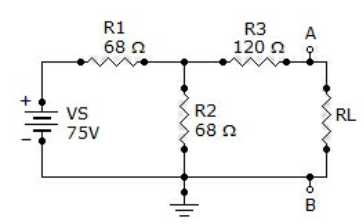(A) 34 Ω
(B) 68 Ω
(C) 120 Ω
(D) 154 Ω

Q 28. A 680 Ω load resistor, RL, is connected across a constant current source of 1.2 A. The internal source resistance, RS, is 12 kΩ. The load current, RL, is

(A) 0 A
(B) 1.2 A
(C) 114 mA
(D) 1.14 A

Q 29. An 18 V source has an internal resistance of 70 Ω. If a load resistance of 33 Ω is connected to the voltage source, the load power, PL, is

(A) 0 W
(B) 1 W
(C) 175 mW
(D) 18 mW

Q 30. What is the Thevenin equivalent (VTH and RTH) for the circuit given?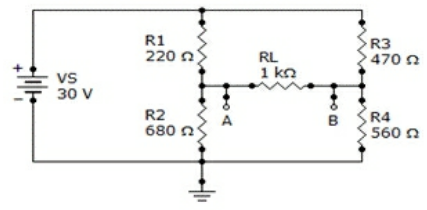(A) 6.4 V, 422 Ω
(B) 6.4 V, 560 Ω
(C) 6.4 V, 680 Ω
(D) 30 V, 422 Ω

Q 31. Find the current through R2 of the given circuit.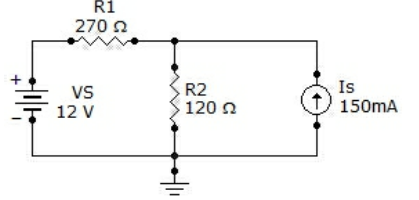(A) 30.7 mA
(B) 104 mA
(C) 74 mA
(D) 134 mA

Q 32. A 120 V voltage source has a source resistance, RS, of 60 Ω. The equivalent current source is

(A) 2 A
(B) 4 A
(C) 200 mA
(D) 400 mA

Q 33. A 120 Ω load is connected across a voltage source with VS = 12 V and RS = 8 Ω. The voltage across the load is

(A) 11.25 V
(B) 0 V
(C) 12 V
(D) 1.13 V

Q 34. Find the total current through R3 in the given circuit.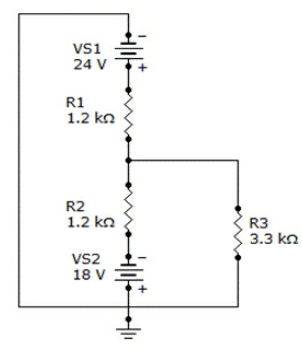(A) 7.3 mA
(B) 5.5 mA
(C) 12.8 mA
(D) 1.8 mA